[BACK]Computer Modeling in Engineering & SciencesDOI: 10.32604/cmes.2021.014896

ARTICLE

Quantile Version of Mathai-Haubold Entropy of Order Statistics

1Department of Mathematics, College of Science, King Khalid University, Abha, 62529, Saudi Arabia
2Statistical Research and Studies Support Unit, King Khalid University, Abha, 62529, Saudi Arabia
3Department of Mathematical Sciences, IUST, Kashmir, 192231, India
4Department of Mathematics, Faculty of Science, Al al-Bayt University, Mafraq, 25113, Jordan
5Department of Mathematics, Govt. Degree College Kilam, Higher Education, J & K, 192231, India
*Corresponding Author: Ibrahim M. Almanjahie. Email: imalmanjahi@kku.edu.sa
Received: 06 November 2020; Accepted: 18 March 2021

Abstract: Many researchers measure the uncertainty of a random variable using quantile-based entropy techniques. These techniques are useful in engineering applications and have some exceptional characteristics than their distribution function method. Considering order statistics, the key focus of this article is to propose new quantile-based Mathai-Haubold entropy and investigate its characteristics. The divergence measure of the Mathai-Haubold is also considered and some of its properties are established. Further, based on order statistics, we propose the residual entropy of the quantile-based Mathai-Haubold and some of its property results are proved. The performance of the proposed quantile-based Mathai-Haubold entropy is investigated by simulation studies. Finally, a real data application is used to compare our proposed quantile-based entropy to the existing quantile entropies. The results reveal the outperformance of our proposed entropy to the other entropies.

Keywords: Shannon entropy; Mathai-Haubold entropy; quantile function; residual entropy; order statistics; failure time; reliability measures

1  Introduction

The order statistics are considered in a varied scope of complicated problems, including characterization of a probability distribution, quality control, robust statistical estimation and identifying outliers, analysis of a censored sample, the goodness of fit-tests, etc. Based on order statistics, the usage of the recurrence relationships for moments is well recognized by many researchers (see, for instance, Arnold et al. , Malik et al. ). For an enhancement, many recurrence relations and identities for the order statistics moments originating from numerous particular continuous probability distributions (i.e., gamma, Cauchy, normal, logistic, and exponential) have been reviewed by Samuel et al.  and Arnold et al. .

Based on a random sample of X1,X2,,Xn, let the corresponding order statistics to be X1:nX2:nXn:n. Then, as in David  and Arnold et al. , the density of Xr:n,1rn, is

fr:n(x)=Cr:n{[F(x)]r1[1F(x)]nrf(x)},0<x<, (1)

with Cr:n=n!(r1)!(nr)!. Eq. (1) can be used to determine the smallest (when r=1) and largest (when r=n) probability density functions; they, respectively, are f(1,n)(x)=n[1F(x)]n1f(x) and f(n,n)(x)=n[F(x)]n1f(x). The corresponding distribution functions are obtained, respectively, by F(1,n)(x)=1[1F(x)]n and F(n,n)(x)=[F(x)]n.

Shannon  was the first author who introduced the entropy idea for a random variable (r.v.) X in the field of information theory and defined it as

H(X)=0fX(x)logfX(x)dx. (2)

Here, fX(x) indicates the pdf of the r.v. X. Based on the Shannon entropy measure, Mathi et al.  (now onwards M-H entropy) developed a generalized version of Eq. (2) and defined it as

Mα(X)=1α1(0[f(x)]2αdx1),0<α<2, α1. (3)

When α1 the M-H entropy measure Mα(X) will be reduced to the Shannon entropy measure defined in Eq. (2).

Mathai et al.  and Sebastian  discussed the main property allied with Eq. (3). In other words, applying the maximum entropy and using its normalization version together with energy restrictions will result in the well-recognized pathway-model as provided by Mathai . However, this model contains many special cases of familiar probability distributions.

Theoretical surveys and applications employing the measurement information are distributional dependents, and they may be found to be not appropriate in circumstances once the distribution is analytically not tractable. Hence, utilizations of quantile function are considered as an alternative method, where

Q(u)=F1(u)=inf{x/F(x)u},0u1.

We refer the readers to Nair et al.  and Sunoj et al.  and references therein for more details about quantile function. Recently, Sunoj et al.  studied Shannon entropy and as well as its residual and introduced quantile versions of them defined as

H=01logq(p)dp (4)

and

H(u)=H(X;Q(u))=log(1u)+11uu1logq(p)dp (5)

respectively, where q(u)=dQ(u)du denotes the density of quantile function. If we define the quantile density function by fQ(u)=f(Q(u)), then, we obtain

q(u)f(Q(u))=1

For Shannon past entropy, Sunoj et al.  also introduced its quantile version and defined it as

H¯(u)=H(X;Q(u))=log(u)+1u0ulogq(p)dp

In the present paper, we work with the order statistics, propose the quantile-based version of M-H entropy and discuss its properties. The M-H divergence measure is also considered and we establish some of its distribution free properties. In addition, we introduce the version of the quantile-based residual for the M-H entropy and prove some characterization results. To the best of our knowledge, the results presented here, treat a research gap that has not been addressed or studied systematically by others, which was the primary motivation of our paper.

The paper is outlined as follows. Section 2 is devoted to the construction of our quantile-based M-H entropy and its properties. Next, expressions for the quantile-based version of M-H entropy for some life-time distributions are presented in Section 3. A quantile-based generalized divergence measure of rth order statistics is given in Section 4. Quantile Residual Entropy of M-H for rth order statistics and also for some lifetime models are introduced in Section 5. Characterization theorems based on M-H Quantile Residual Entropy are presented in Section 6. In Section 7, simulation studies for investigating the performance of our proposed quantiles and real data life application are presented. Our conclusion is stated in Section 8.

2  Quantile Based M-H Entropy of rth Order Statistics

Wong et al. , Park , Ebrahimi et al.  and Baratpour et al.  are the authors who discuss in detail the aspects of information-theoretic based on order statistics. Paul et al.  considered the M-H entropy and, based on record values, studied some of its essential properties. For rth order statistics Xr;n, the M-H entropy is defined as

Mα(Xr;n)=1α1(0[fr;n(x)]2αdx1),0<α<2, α1,

where fr;n(x) is given in Eq. (1). Now, FQ(u)=u, then, the pdf of rth order statistics becomes

fr;n(u)=fr;n(Q(u))=1β(r,nr+1)ur1(1u)nrf(Q(u))=gr(u)q(u),

where gr(u) denotes beta-distribution density with r and (nr+1) as its parameters. The quantile-based M-H entropy of Xr;n is determined by

MXr:nα=MXr:nα(Q(u))=1α1(01fr:n2α(Q(u))d(Q(u))1)=1α1(01(gr(u))2α(q(u))α1du1) (6)

Remark 2.1: For α1, Eq. (6) reduces to

MXr:n=01gr(u)loggr(u)q(u)du,

which is the quantile entropy of rth order statistics investigated by Sunoj et al. .

3  Expressions for Some Distributions

In the following, we provide expressions for Quantile-based M-H entropy of order statistics for some life time distributions:

(i) Govindarajulu’s Distribution: The quantile version and the corresponding density functions, respectively, are

Q(u)=a{(b+1)ubbub+1} and q(u)=ab(b+1)(1u)ub1,0u1; a,b>0.

Using Eq. (6), we can easily obtain quantile-based M-H Entropy of rth order statistics for Govindarajulu distribution as

MXr:nα=1α1{[(ab)(b+1)]α1β(r(2α)+b(α1),(nr)(2α)+α)(β(r,nr+1))2α1}.

Similarly, based on the quantile and quantile density functions, we obtain the quantile-based for the M-H Entropy (MXr:nα) of rth order statistics for the following distributions.

(ii) Uniform Distribution:

Q(u)=a+(ba)u and q(u)=(ba),0u1; a<b.

MXr:nα=1α1{(ba)α1β((r1)(2α)+1,(nr)(2α)+1)(β(r,nr+1))2α1}.

(iii) Pareto-I Distribution:

Q(u)=b{(1u)1a} and q(u)=ba{(1u)(1+1a)},0u1; a,b>0.

MXr:nα=1α1{(ba)α1β((r1)(2α)+1,(nr)(2α)+1αaα+2aa)(β(r,nr+1))2α1}.

(iv) Exponential distribution:

Q(u)=log(1u)λ and q(u)=1λ(1u),0u<1; λ>0.

MXr:nα=1α1{β((r1)(2α)+1,(nr)(2α)+α1)λ2α(β(r,nr+1))2α1}.

(v) Power distribution

Q(u)=au1b and q(u)=abu1b1,0u1; λ>0.

Mα(Xr;n,u)=1α1{(ab)(α1)β((r1)(2α)(α1)+1/b(α1)α+2;(nr)(2α)+1)(β(r,nr+1))2α1}

Figs. 13 give the quantile version of M-H entropy plots of smallest order statistics under exponential, Pareto-I and uniform distributions, respectively.Figure 1: Quantile M-H entropy plots of smallest order statistics (Exponential distribution)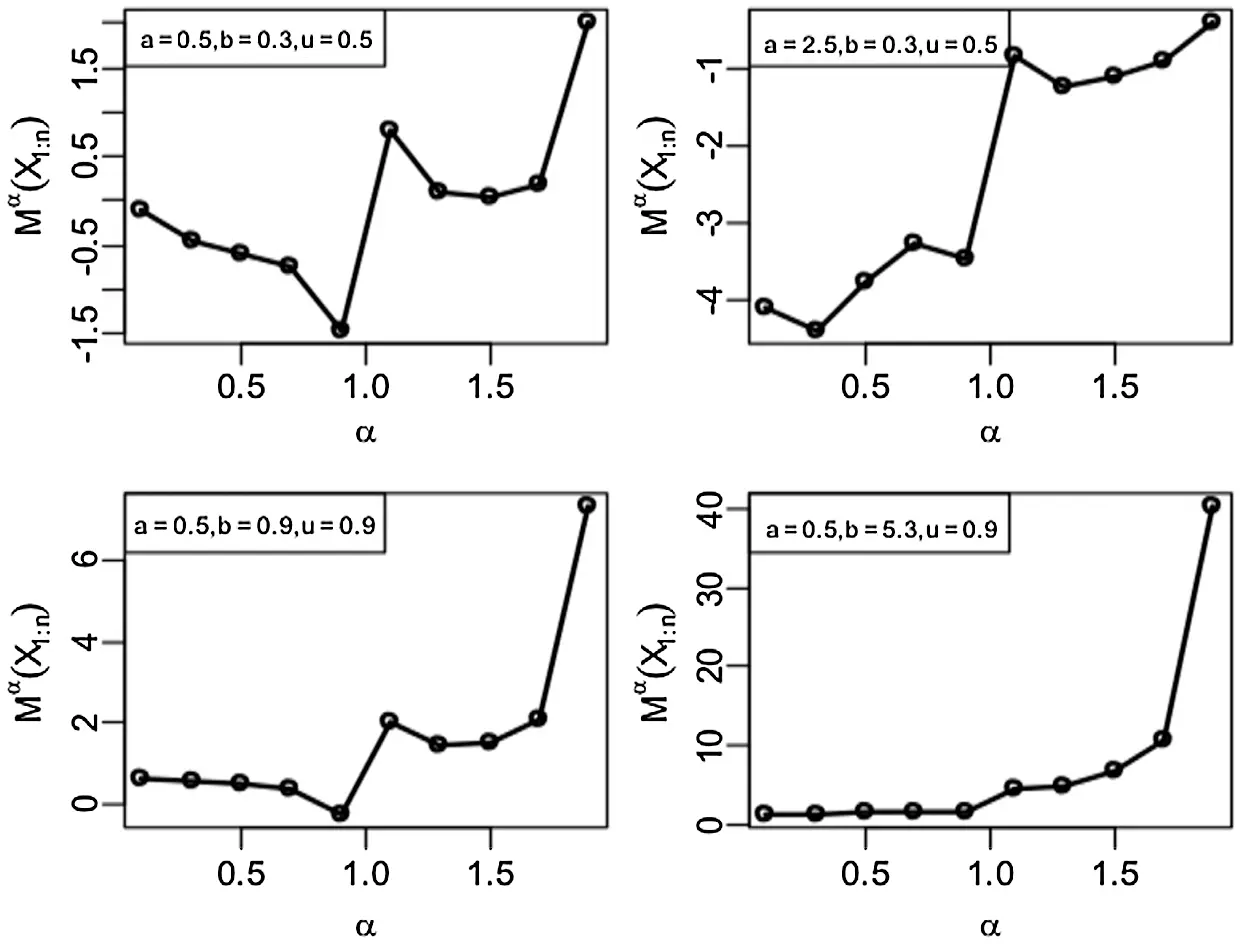Figure 2: Quantile M-H entropy plots of smallest order statistics (Pareto-I Distribution)

For an increasing value of parameters α and λ, the entropy plot, based on the exponential distribution, increases. In the case of entropy plot under the Pareto-I distribution, the plot has an increasing (a decreasing) behaviour for different parameter combinations. The entropy plot under uniform distribution also has increasing behaviour for different parameter values. Tabs. 1--3 give entropy values when the parameters α and λ are varied.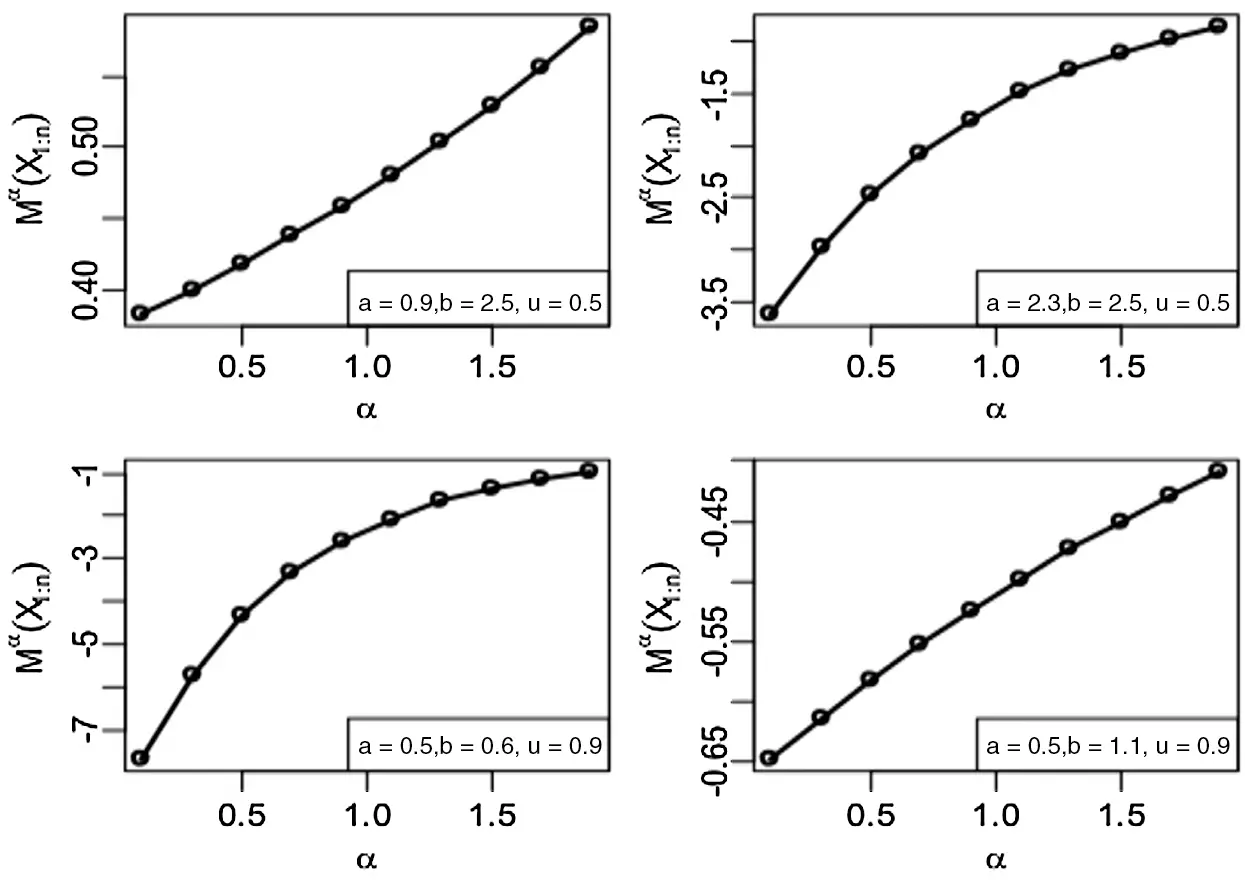Figure 3: Quantile M-H entropy plots of smallest order statistics (Uniform Distribution)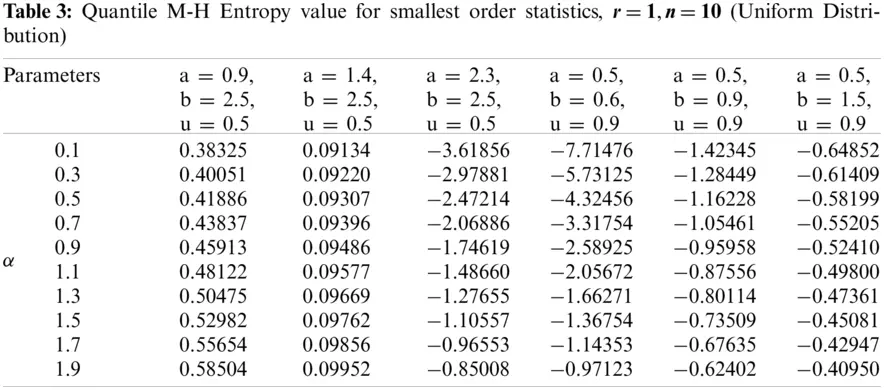Clearly, we see from Tabs. 13 that the entropy values under exponential, Pareto-I and uniform distributions portray the same behaviour as discussed in the graphical plots.

4  Quantile-Based Generalized Divergence Measure of rth Order Statistics

Different measures deal with the dissimilarity or the distance between two probability distributions. Certainly, these measures are essential in theory, inferential statistics, applied statistics and data processing sciences, such as comparison, classification, estimation, etc.

Assume f and g are the density functions of the non-negative r.vs X and Y, respectively. The direct divergence of f from g is measured by the Kullback et al.  and is

D(f/g)=0f(x)logf(x)g(x)dx. (7)

With order α, the divergence measure of the M-H or the relative-entropy of g concerning f is obtained by

Mα(f/g)=11α{0f(x)(f(x)g(x))1αdx1}, (8)

where, for α1, the expression in Eq. (8) reduces to Eq. (7) (see Kullback et al. ).

Theorem 4.1: The quantile-based-generalized divergence measure between the rth order statistics distribution and the primary distribution is a distribution-free.

Proof: From equation Eq. (8), we have

Mα(Xr:n/X)=11α{0(fr:n(x)f(x))1αfr:n(x)dx1}. (9)

Now, using the value of fr:n(x) in Eq. (9), we obtain Mα(Xr:n/X)=11α{0F(2α)(r1)(x)(1F(x))(nr)(2α)(β(r,nr+1))2αf(x)dx1}.

Using the fact that q(u)f(Q(u))=1, we determine the quantile-based-generalized divergence measure between the distribution of rth order statistics and primary distribution, as

Mα(Xr:n/X)=11α{β((3α)(r1)+1),(nr)(3α)+1)(β(r,nr+1))3α1}

which is a distribution-free. Hence, the theorem is proved.

5  M-H Quantile Residual Entropy for rth Order Statistics

Entropy functions are very popular in the applications of finance and tectonophysics, machine learning, reliability theory, etc. However, in reliability and real-life applications, the life test time is truncated at a specific time, and in such situations, Eq. (2) is not an appropriate measure. Therefore, Shannon's entropy is not an adequate measure when we have knowledge about the component's current age, which can be used when determining its uncertainty. Ebrahimi  describes a more practical approach that considers the use of age, defined as

H(X;t)=tf(x)F¯(t)logf(x)F¯(t)dx,

with F¯(t) indicates the survival-function. Note that for t=0, the Eq. (5) reduces to Eq. (4). Denote by X, a non-negative r.v., the unit’s life-time at time t. Then, the residual function of M-H  is

Mα(X;t)=1α1{tf2α(x)F¯2α(t)dx1},0<α<2, α1.

The M-H residual entropy for the rth order statistics is given by

Mα(Xr;n;t)=1α1{tfr;n2α(x)dx(F¯r;n(t))2α1},t0,

where F¯r;n(x)=β¯x(r,nr+1)β(r,nr+1) refers to the survival-function of the rth order statistics and β¯x(r,nr+1) is the incomplete gamma function.

Considering the rth order statistic, the quantile residual entropy function of M-H of is given by

Mα(Xr;n;u)=Mα(Xr;n;Q(u))=1α1{(β(r,nr+1))2α(β¯u(r,nr+1))2αu1gr2α(p)qα1(p)dp1}. (10)

The following theorem will state important result.

Theorem 5.1: Considering the rth order statistic, the quantile residual entropy function of M-H determines the underlying distribution uniquely.

Proof: Using equation Eq. (10), we obtain

( α1 ) ( β ¯ u ( r,nr+1 ) ) 2α M α ( X r;n ;u )= u 1 p ( 2α )( r1 ) ( 1p ) ( 2α )( nr ) (q(p)) α1 dp ( β ¯ u (r,nr+1)) 2α

Differentiate both sides with respect to (w.r.t) u to obtain

(q(u))α1=ur1((1u)nr)(β¯u(r,nr+1))1αu(2α)(r1)(1u)(2α)(nr){(2α)+(2α)(α1)Mα(Xr;n;u)}(α1)(β¯u(r,nr+1))2αu(2α)(r1)(1u)(2α)(nr)(Mα(Xr;n;u)),

where denotes the differentiation w.r.t u. This equation involved an immediate connection between the q(u) and Mα(Xr;n;u), which implies that the quantile residual entropy function of M-H of rth order statistic leads to the unicity of the underlying distribution.

Next, we make the derivation of the quantile form of M-H residual entropy of the rth order statistic for some lifetime models.

(i) Govindarajulu’s Distribution

The quantile for the Govindarajulu distribution is

Q(u)=a{(b+1)ubbub+1},

and the corresponding density is

q(u)=ab(b+1)(1u)ub1,0u1; a,b>0.

The quantile residual entropy function of M-H of rth order statistics for the distribution of Govindarajulu is

M α ( X r;n ,u )= 1 α1 ×{ [ ( ab )( b+1 ) ] α1 β ¯ u ( ( r1 )( 2α )( α1 )( b1 )+1;( nr )( 2α )( α1 )+1 ) ( β ¯ u ( r,nr+1 ) ) 2α 1 }.

Similarly, based on the quantile and quantile density functions, we obtain the quantile-based residual M-H Entropy of rth order statistics for the following distributions.

(ii) Uniform Distribution Q(u)=a+(ba)u and q(u)=(ba),0u1; a<b.

Mα(Xr;n,u)=1α1{(ba)α1β¯u((r1)(2α)+1;(nr)(2α)+1)(β¯u(r,nr+1))2α1}.

(iii) Pareto-I Distribution

Q(u)=b{(1u)1a} and q(u)=ba{(1u)(1+1a)},0u1; a,b>0

Mα(Xr;n,u)=1α1{(ba)α1β¯u((r1)(2α)(α1)+1;(nr)(2α)α(α1a)+2)(β¯u(r,nr+1))2α1}.

(iv) Exponential distribution Q(u)=log(1u)λ and q(u)=1λ(1u),0u<1; λ>0.

MXr:nα=1α1{β¯u((r1)(2α)+1,(nr)(2α)α+2)λ2αβ¯u((r,nr+1))2α1}.

(v) Power distribution Q(u)=au1b and q(u)=abu1b1,0u1; λ>0.

Mα(Xr;n,u)=1α1{(ab)α1β¯u((r1)(2α)(α1)+1/b(α1)α+2;(nr)(2α)+1(β¯u(r,nr+1))2α1}.

Based on residual M-H quantile entropy of order statistics Mα(Xr;n,u), the following nonparametric classes of life distribution are defined.

Definition 5.1: X is said to have an increasing (a decreasing) M-H quantile entropy of order statistics if Mα(Xr;n,u) is increasing (decreasing) in u0.

The following lemma is useful in proving the results in monotonicity of Mα(Xr;n,u).

Lemma 5.1: Let f(u,x):R+2R+ and g:R+R+ be any two functions. If uf(u,x)dx is increasing and g(u) is increasing (decreasing) in u, then uf(u,x)g(x)dx is increasing (decreasing) in u, provided the existence of integrals.

Theorem 5.2: Let X be a non-negative and continuous r.v. with quantile QX(.) and density qX(.). Define Y=(X), where (.) is nonnegative, increasing and convex(concave) function. Then,

(i) For 1<α<2, Mα(Yr;n,u) increases (or decreases) in u whenever Mα(Xr;n,u) increases (or decreases) in u.

(ii) For 0<α<1, Mα(Yr;n,u) increases (or decreases) in u whenever Mα(Xr;n,u) increases (or decreases) in u.

Proof: (i) The quantile density of Y is given by g(QY(u))=1qY(u)=1qX(u)(QX(u)).

Thus, we have

Mα(Yr;n;u)=1α1{(β(r,nr+1))2α(β¯u(r,nr+1))2αu1gr2α(p)(qX(p)(QX(p)))α1dp1} (11)

From the given condition,Mα(Xr;n,u) is increasing in u, therefore,

{(β(r,nr+1))2α(β¯u(r,nr+1))2αu1gr2α(p)(qX(p))α1dp1}

is increasing in u.

Since 1<α<2 and is non-negative, increasing and convex (concave) function, the ((QX(p))α1 increases (or decreases) and it is also non-negative. Consequently, using Lemma 3.1, Eq. (11) is increasing (decreasing), which gives the proof of (i) of the Theorem. Similarly, 0<α<1, ((QX(p))α1 increases (or decreases) in p, because increases and it is convex. Consequently, Eq. (11) is decreasing (increasing) in u, which proves (ii) of the Theorem. The immediate application of Theorem 5.2 is given below:

Let X be an r.v. following the distribution of exponential and having a failure rate λ. Also, let Y=X1α,α>0. Therefore, Y follows Weibull distribution where Q(u)=λ1α(log(1u))1α. The function ϕ(x)=x1α,x>0,α>0 is a convex (concave) if 1<α<2,(0<α<1). Hence, based on Theorem 5.2, the Weibull distribution is increasing (decreasing) M-H quantile entropy of order statistics if 1<α<2,(0<α<1).

6  Characterization Theorems Based on M-H Quantile Residual Entropy

This section provides some characterizations for the quantile M-H residual entropies of the smallest and largest order statistics. The corresponding quantile M-H residual entropy can be determined by substituting r=1 (for smallest) and r=n (for largest) in Eq. (10) and are, respectively, given by

Mα(X1;n;u)=Mα(X1;n;Q(u))=1α1{n2α(1u)n(2α)u1g12α(p)qα1(p)dp1} (12)

Mα(Xn;n;u)=Mα(Xn;n;Q(u))=1α1{n2α(1un)(2α)u1gn2α(p)qα1(p)dp1}.

Now, we define the hazard and the reversed hazard functions for the quantile version which are, respectively, corresponding to the well-recognized hazard rate and reversed hazard rate functions, as K(u)=h(Q(u))=fQ(u)(1u)=1(1u)q(u) and K¯(u)=K¯(Q(u))=fQ(u)F(Q(u))=(uq(u))1.

In numerous practical circumstances, the uncertainty is essentially not identified with the future. Therefore, it can likewise allude to the past. This thought empowered Crescenzo et al.  to build up the idea of past entropy on (0,t). If X denotes the life-time of a component, thus, the past entropy of X is obtained by

H0(X;t)=0tf(x)F(t)logf(x)F(t)dx, (13)

with F(t) is the cumulative distribution function. For t=0, Eq. (13) reduces to Eq. (2).

The quantile form of past M-H residual entropy of rth order statistic is determined by

M¯α(Xr;n;u)=M¯α(Xr;n;Q(u))=1α1{1(β¯u(r,nr+1))2α0ugr2α(p)qα1(p)dp1}.

For sample maxima Xn;n, the past quantile entropy of M-H is given by

M¯α(Xn;n;u)=1α1{n2(2α)(u)n(2α)0up(2α)(n1)qα1(p)dp1}. (14)

Next, we state some properties based on quantile M-H residual entropy of the smallest order statistics.

Theorem 6.1: Let X1;n represents the first order statistics with survival and hazard quantile functions F¯1;n(x) and KX1;n(u). Then, the Mα(X1;n;u) is determined by

Mα(X1;n;u)=1α1{C(KX1;n(u))1α1},0<α<2,α1, (15)

if and only if:

(i) X follows an exponential distribution, if C=12α.

(ii) X follows Pareto distribution where the quantile density function is q(u)=ba{(1u)(1+1a)},0u1; a,b>0 if C<12α.

(iii) X follows a finite range distribution with quantile density function q(u)=ba{(1u)(1a1)},0u1; b>0,a>1 if C>12α.

Proof: Assume that the conditions in Eq. (15) are held. Then, using Eq. (12), we have

n2α(1u)n(2α)u1(1p)(2α)(n1)qα1(p)dp=C(KX1;n(u))1α. (16)

Therefore, using KX1;n(u)=n(1u)q(u) in Eq. (16), then, differentiating (w.r.t) u, we obtain

q(u)q(u)=(2ncncα+αccnccα)(11u).

This implies q(u)=A(1u)n(2ccα1cαc1), where A is a constant. Thus, X has exponential distribution, Pareto distribution and finite range distribution if C=12α,C<12α,C>12α, respectively.

Theorem 6.2: For the exponential distribution, the difference between quantile-based M-H residual entropy of the life-time of the series system (Mα(X1;n;u)) and M-H residual quantile entropy of the life-time of each component (Mα(X;u)) is independent of u and relies only on α and the number of components of the system.

Proof: For the exponential distribution, we have

Mα(X1;n;u)=1α1((nλ)1αα1) and Mα(X;u)=1α1((λ)1αα1).

Therefore,

Mα(X1;n;u)Mα(X;u)=(λ1αα(α1))(n1α1),

which complete the prove of Theorem 6.2.

Theorem 6.3: Let Xn;n represents the largest order statistics with hazard and survival quantile functions, K¯Xn;n(u) and F¯n;n(x). Then, for sample maxima Xn;n, the past quantile entropy of M-H, M¯α(Xn;n;u), is

M¯α(Xn;n;u)=1α1{C(K¯Xn;n(u))1α1},0<α<2, (17)

if and only if X follows the power distribution.

Proof: The quantile and quantile density functions for the power distribution are, respectively,

Q(u)=au1b and q(u)=abu1b1,0u1; a,b>0.

It is simple to show that K¯Xn;n(u)=nbu1ba. Taking C=bn3α2nbnbα+α1 gives the if part of Theorem.

Conversely, let Eq. (17) is valid. Thus, using Eq. (14), we determine

{n2(2α)(u)n(2α)0up(2α)(n1)qα1(p)dp}=C(K¯Xn;n(u))1α.

Substituting K¯Xn;n(u)=nuq(u), we have {n3α0up(2α)(n1)qα1(p)dp}=Cu2nnα+α1(q(u))α1.

Taken the derivative (w.r.t) u yields q(u)q(u)=(n3αC(2nnα+α1)CαC)(1u). The latter gives

q(u)=Au(n3αC(2nnα+α1)C(α1)),

where A denotes a constant. Hence, the power distribution is a characterized for C=bn3α2nbnbα+α1.

7  Simulation Study and Application to Real Life Data

In this paper, the quantile-based M-H entropy is proposed for some distributions. However, based on the available real data and to keep the simulation study related to the application part, we investigate the performance of the quantile-based M-H entropy for the exponential distribution.

7.1 Simulation Study

We conducted simulation studies to investigate the efficiency of the quantile-based M-H entropy estimators of smallest order statistics for exponential distribution (MX1:nα) in terms of the average bias (Bias), variance and mean squared error (MSE), based on sample sizes 10, 25, 100, 200 and 500 for different parameter combinations. The estimation of parameter λ was achieved using ML estimation and the process was repeated 2000 times.

From the results of the simulation study (see Tabs. 4 and 5), conclusions are drawn regarding the behaviour of the entropy estimator in general, which are summarized below:

1.    The ML estimates of MX1:nα approaches to true value when sample size n increases.

2.    When sample size n is increased, the MSE and variance of MX1:nα decreases.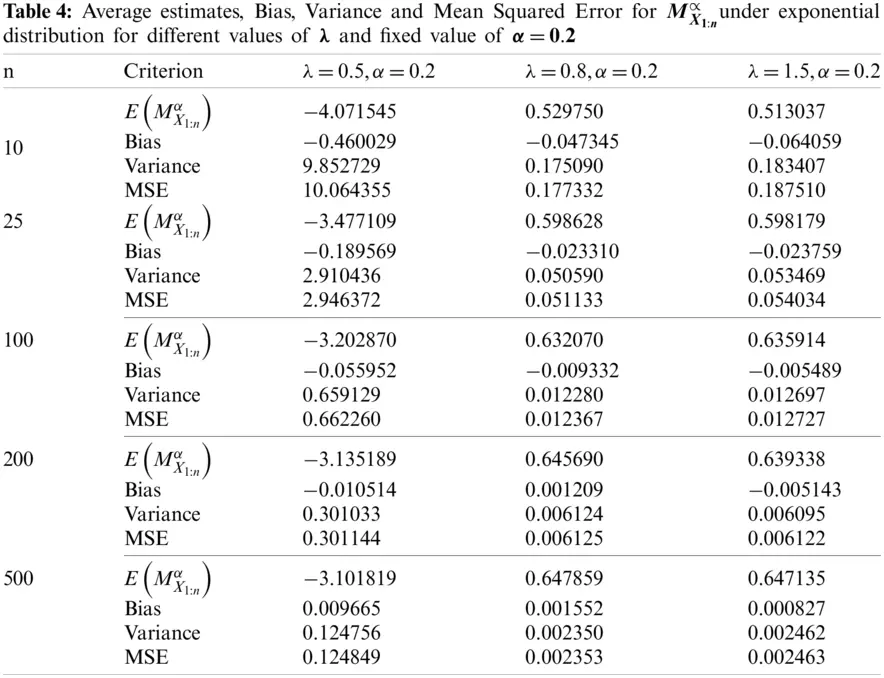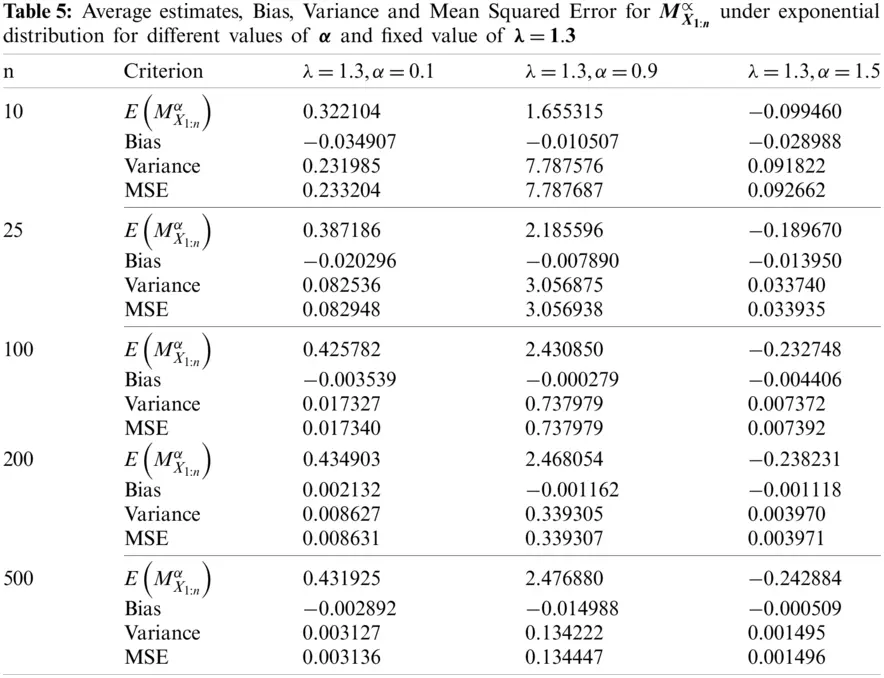7.2 Application to Real Life Data

The real data in this section represents the failure times of 20 mechanical components that were used previously by Murthy et al.  for investigating some of Weibull models. The data values are: 0.067, 0.068, 0.076, 0.081, 0.084, 0.085, 0.085, 0.086, 0.089, 0.098, 0.098, 0.114, 0.114, 0.115, 0.121, 0.125, 0.131, 0.149, 0.160, 0.485. We use this data for two main purposes: (i) for investigating the performance of our quantile-base M-H entropy (MX1:nα) in the exponential distribution case, and (ii) for comparing MX1:nαto the quantile-based Tsallis entropy (HX1:nα) that was proposed by Kumar .

Based on this data, we used first the maximum likelihood method to estimate the exponential distribution parameter, λ^=0.122. Then, for different values of α, varied from 0.1 to 0.9, we calculate the estimated values of MX1:nα and HX1:nαof smallest order statistics under exponential distribution. The results are displayed in Tab. 6.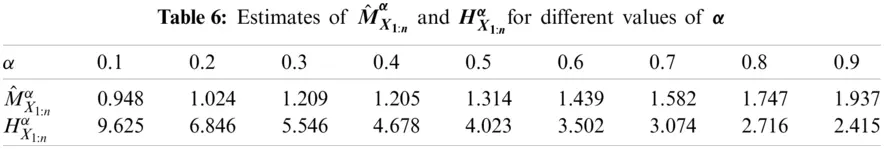It should be noted that the estimated values of M^X1:nαis generally increased when 0<α<1. Also, the results in Tab. 6 indicate clearly that the estimated entropy values based on M^X1:nαare less than those given by HX1:nα.

8  Conclusion

The key focus of this article is to propose new quantile-based Mathai-Haubold entropy and investigate its characteristics. We also considered the divergence measure of the Mathai-Haubold and established some of its properties. Further, based on order statistics, we propose the residual entropy of the quantile-based Mathai-Haubold and some of its property results are proved. The performance of the proposed quantile-based Mathai-Haubold entropy is investigated by simulation studies and also by using real data application example. The proposed quantile-based Mathai-Haubold entropy's performance is investigated by simulation studies and by using a real data application example. We found that the ML estimates of MX1:nα approach true value when sample size n increases for the simulation part. For the application part, we compared our proposed quantile-based entropy to the existing quantile entropies and the results showed the outperformance of our proposed entropy to the other entropies. Our proposed quantile-based Mathai-Haubold entropy is useful for many future engineering applications such as reliability and mechanical components analysis.

Acknowledgement: The authors would like to thank the anonymous reviewers and the editor for their useful suggestions and comments which increase and improve the quality of this paper.

Funding Statement: Authors thank and appreciate funding this work by the Deanship of Scientific Research at King Khalid University through the Research Groups Program under the Grant No. (R.G.P. 2/82/42).

Conflicts of Interest: The authors declare that they have no conflicts of interest to report regarding the present study.

## References

1. Arnold, B. C., Blakrishan, N., Nagraja, N. H. (1992). A first course in order statistics. New York: John Wiley and Sons.
2. Malik, J. H., Balakrishnan, N., & Ahmad, S. E. (1998). Recurrence relations and identities for moments of order statistics I: Arbitrary continuous distributions. Communication in Statistics–Theory and Methods, 17(8), 2623-2655. [Google Scholar] [CrossRef]
3. Samuel, P., & Thomas, P. Y. (2000). An improved form of a recurrence relation on the product moment of order statistics. Communication in Statistics-Theory and Methods, 29(7), 1559-1564. [Google Scholar] [CrossRef]
4. David, H. A. (1981). Order statistics. Second Edition. New York: John Willey and Sons.
5. Shannon, C. E. (1948). A mathematical theory of communication. Bell System Technical Journal, 27, 379-423. [Google Scholar] [CrossRef]
6. Mathai, A., & Haubold, H. (2007). On generalized entropy measures and pathways. Physica A: Statistical Mechanics and its Applications, 385(2), 493-500. [Google Scholar] [CrossRef]
7. Sebastian, N. (2015). Generalized pathway entropy and its applications in diffiusion entropy analysis and fractional calculus. Communications in Applied and Industrial Mathematics, 6(2), 1-20. [Google Scholar] [CrossRef]
8. Mathai, A. (2005). A pathway to matrix-variate gamma and normal densities. Linear Algebra and its Applications, 396, 317-328. [Google Scholar] [CrossRef]
9. Nair, N. U., & Sankaran, P. G. (2009). Quantile-based reliability analysis. Communication in Statistics-Theory and Methods, 38, 222-232. [Google Scholar] [CrossRef]
10. Sunoj, S. M., Krishnan, A. S., & Sankaran, P. G. (2017). Quantile-based entropy of order statistics. Journal of the Indian Society for Probability and Statistics, 18(1), 1-17. [Google Scholar] [CrossRef]
11. Sunoj, S. M., & Sankaran, P. G. (2012). Quantile based entropy function. Statistics and Probability Letters, 82(6), 1049-1053. [Google Scholar] [CrossRef]
12. Wong, K. M., & Chen, S. (1990). The entropy of ordered sequences and order statistics. IEEE Transaction Information Theory, 36, 276-284. [Google Scholar] [CrossRef]
13. Park, S. (2007). The entropy of consecutive order statistics. IEEE Transaction Information Theory, 41(6), 2003-2007. [Google Scholar] [CrossRef]
14. Ebrahimi, N. (1996). How to measure uncertainty in the residual lifetime distribution. Sankhya SerA, 58, 48-56. [Google Scholar]
15. Baratpour, S., Ahmadi, J., & Arghami, N. R. (2007). Some characterizations based on entropy of order statistics and record values. Communications in Statistical-Theory and Methods, 36, 47-57. [Google Scholar] [CrossRef]
16. Paul, J., & Yageen, P. (2019). On some properties of Mathai-Haubold entropy of record values. Journal of the Indian Society for Probability and Statistics, 20(1), 31-49. [Google Scholar] [CrossRef]
17. Kullback, S., & Leibler, R. A. (1951). On information and sufficiency. Annals of Mathematical Statistics, 22, 79-86. [Google Scholar] [CrossRef]
18. Dar, J. G., & Al-Zahrani, B. (2013). On some characterization results of life time distributions using Mathai-Haubold residual entropy. International Organization of Scientific Research, 5(4), 56-60. [Google Scholar] [CrossRef]
19. Crescenzo, A. D., & Longobardi, M. (2002). Entropy based measure of uncertainty in past lifetime distribution. Journal of Applied probability, 39(2), 434-440. [Google Scholar] [CrossRef]
20. Murthy, D. N. P., Xie, M., Jiang, R. Y. (2004). Weibull models. USA: John Wiley & Sons.
21. Kumar, V. R. (2018). A quantile approach of Tsallis entropy for order statistics. Physica A: Statistical Mechanics and its Applications, 503, 916-928. [Google Scholar] [CrossRef]
22. Kumar, V., & Singh, N. (2019). Quantile-based generalized entropy of order (α, β) for order statistics. Statistica, 78(4), 299-318. [Google Scholar] [CrossRef]
23. Nisa, R., & Baig, M. A. K. (2019). Characterization results based on quantile version of two parametric generalized entropy of order statistics. Journal of Probability and Mathematical Statistics, 1, 1-17. [Google Scholar] [CrossRef]
24. Qiu, G. (2019). Further results on quantile entropy in the past lifetime. Probability in the Engineering and Informational Sciences, 33(1), 149-159. [Google Scholar] [CrossRef]
25. Krishnan, A. S. (2019). A study on some information measures using quantile functions (Ph.D. Thesis). Cochin University of Science and Technology, India.This work is licensed under a Creative Commons Attribution 4.0 International License, which permits unrestricted use, distribution, and reproduction in any medium, provided the original work is properly cited.